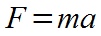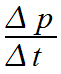This is the time rate change in momentum. It is the change in momentum divided by the change in time, or delta p over delta t.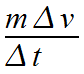Substitution: The change in momentum is equal to mass times the change in velocity, or delta p is equal to m times delta v.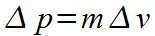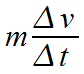The factors are grouped so that we can clearly see that delta v is divided by delta t.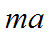Substitution: The change in velocity divided by the change in time is the acceleration. Delta v divided by delta t is a.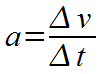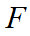Substitution: The mass of an object times its acceleration is equal to the net force applied to the object.